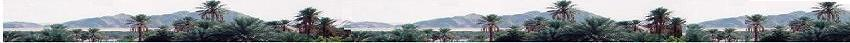Contents
 • one choice • many choices: permutations • many choices: combinations • many choices: combinations • independent events • dependent events • permutations without repettitions • permutations with identical elements • combinations • coditional probabilities • and or events • two outcomes • total probability theorem • Bayes rule • union sets and probability • fundamental counting principle

 Other exercices

 Combinatorics - Probability
Probability & StatisticsToday: : ____________

calculator for combinatorics probability and Statistics